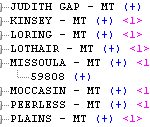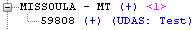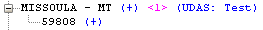# MDX and ASO – Implied Share Metadata Oddities

#### Essbase is Wrong

Once or twice I’ve got stuck troubleshooting an ASO query or MDX member formula, only to discover – after some head-scratching – that Essbase and I disagree about the appropriate value of a metadata property on an implicitly shared member. Whether the (very strange) behavior described below is by design I don’t know, but since ASO and BSO do not behave the same way and I can’t find any documentation describing a difference in this area, I have to suspect not.

The problem is also easily fixable, either on a case-by-case basis with “Never Share”, or by disabling implied sharing globally with the IMPLIED_SHARE Essbase.cfg file setting. So I’m presenting it here more as a curiosity than for in-depth “best practice” advice purposes.  That said, it doesn’t hurt to be able to recognize this problem immediately if you run into it.

#### Level Check

Here’s a snippet of the ASOsamp.Sample Geography dimension:I picked Missoula, MT because it only has one ZIP code in the outline, which means that there is an implied share relationship between [MISSOULA – MT] and ZIP code .   is at level-0 and [MISSOULA – MT] at level-1.  This can be confirmed with a couple of MDX queries using the LEVEL_NUMBER intrinsic property:

```SELECT {} ON AXIS(0),
{[MISSOULA - MT]} DIMENSION PROPERTIES [Geography].[LEVEL_NUMBER] ON AXIS(1)
FROM ASOsamp.Sample;

Axis-1              Axis-1.properties
+-------------------+-------------------
(MISSOULA - MT)     (LEVEL_NUMBER = 1,

SELECT {} ON AXIS(0),
{} DIMENSION PROPERTIES [Geography].[LEVEL_NUMBER] ON AXIS(1)
FROM ASOsamp.Sample;

Axis-1              Axis-1.properties
+-------------------+-------------------
(59808)             (LEVEL_NUMBER = 0,
```

But in MDX there’s almost always more than one way to do things, and this is where the results start to get surprising. The following query uses a calculated measure that tests the level of each  Geography member with the IsLevel function.  The formula returns “1” if the current Geography member is Level-0, and “0” otherwise:

```WITH MEMBER [Measures].Geography_IsLevel0 AS 'IIF(IsLevel([Geography].CurrentMember, 0), 1, 0)'
SELECT {[Measures].Geography_IsLevel0} ON AXIS(0),
{[MISSOULA - MT]} ON AXIS(1)
FROM ASOsamp.Sample;

Axis-1              (Geography_IsLevel0
+-------------------+-------------------
(MISSOULA - MT)                       1

WITH MEMBER [Measures].Geography_IsLevel0 AS 'IIF(IsLevel([Geography].CurrentMember, 0), 1, 0)'
SELECT {[Measures].Geography_IsLevel0} ON AXIS(0),
{} ON AXIS(1)
FROM ASOsamp.Sample;

Axis-1              (Geography_IsLevel0
+-------------------+-------------------
(59808)                               1
```

Huh?  According to the IsLevel function, both the parent and its child are at Level-0, which can’t be true (can it?). And the IsLeaf function behaves in the same way, returning ‘True’ for both [MISSOULA – MT] and :

```WITH MEMBER [Measures].Geography_IsLeaf AS 'IIF(IsLeaf([Geography].CurrentMember), 1, 0)'
SELECT {[Measures].Geography_IsLeaf} ON AXIS(0),
{[MISSOULA - MT]} ON AXIS(1)
FROM ASOsamp.Sample;

Axis-1              (Geography_IsLeaf)
+-------------------+-------------------
(MISSOULA - MT)                       1

WITH MEMBER [Measures].Geography_IsLeaf AS 'IIF(IsLeaf([Geography].CurrentMember), 1, 0)'
SELECT {[Measures].Geography_IsLeaf} ON AXIS(0),
{} ON AXIS(1)
FROM ASOsamp.Sample;

Axis-1              (Geography_IsLeaf)
+-------------------+-------------------
(59808)                               1
```

It’s very easy to envisage situations in which this will create problems if IsLevel or IsLeaf is used to drive MDX formula logic!

#### Just Getting Started…

On further investigation,  level identification is not the only case where properties get confused by an implied share.  I added a UDA, “Test”, to the Geography dimension and applied it to the child member :The IsUDA function returns “True” if the specified member carries a particular UDA.  The following queries indicate that the UDA is carried by both  and [MISSOULA – MT]:

```WITH MEMBER [Measures].Geography_IsUDA_Test AS 'IIF(IsUDA([Geography].CurrentMember, "Test"), 1, 0)'
SELECT {[Measures].Geography_IsUDA_Test} ON AXIS(0),
{} ON AXIS(1)
FROM ASOsamp.Sample;

Axis-1              (Geography_IsUDA_Te
-------------------+-------------------
(59808)                               1

WITH MEMBER [Measures].Geography_IsUDA_Test AS 'IIF(IsUDA([Geography].CurrentMember, "Test"), 1, 0)'
SELECT {[Measures].Geography_IsUDA_Test} ON AXIS(0),
{[MISSOULA - MT]} ON AXIS(1)
FROM ASOsamp.Sample;
Axis-1              (Geography_IsUDA_Te
-------------------+-------------------
(MISSOULA - MT)                       1```

Perhaps the strangest result of all is generated when the “Test” UDA is applied to [MISSOULA – MT] rather than :```WITH MEMBER [Measures].Geography_IsUDA_Test AS 'IIF(IsUDA([Geography].CurrentMember, "Test"), 1, 0)'
SELECT {[Measures].Geography_IsUDA_Test} ON AXIS(0),
{} ON AXIS(1)
FROM ASOsamp.Sample;

Axis-1              (Geography_IsUDA_Te
-------------------+-------------------
(59808)                               0

WITH MEMBER [Measures].Geography_IsUDA_Test AS 'IIF(IsUDA([Geography].CurrentMember, "Test"), 1, 0)'
SELECT {[Measures].Geography_IsUDA_Test} ON AXIS(0),
{[MISSOULA - MT]} ON AXIS(1)
FROM ASOsamp.Sample;
Axis-1              (Geography_IsUDA_Te
-------------------+-------------------
(MISSOULA - MT)                       0```

This time, IsUDA reports that neither member has the “Test” UDA attached, despite the fact that it is clearly visible in the outline viewer and member properties in EAS.  From the point of view of MDX functions, the UDA has simply vanished!  Again, it’s easy to imagine this creating problems, since UDAs are frequently used to drive conditional logic in MDX.